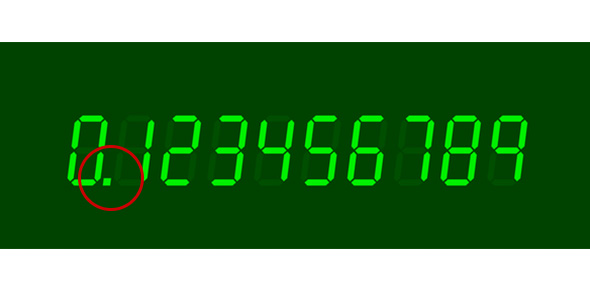# Multiplying Decimals

Approved & Edited by ProProfs Editorial Team
At ProProfs Quizzes, our dedicated in-house team of experts takes pride in their work. With a sharp eye for detail, they meticulously review each quiz. This ensures that every quiz, taken by over 100 million users, meets our standards of accuracy, clarity, and engagement.
| Written by Thickey1
T
Thickey1
Community Contributor
Quizzes Created: 1 | Total Attempts: 3,369
Questions: 5 | Attempts: 3,372Settings6th Grade Math quiz on multiplying multi-digit decimals.

• 1.

### 4.65 X 5.76

• A.

347.92

• B.

267.84

• C.

34.792

• D.

26.784

D. 26.784
Explanation
The correct answer is 26.784 because when you multiply 4.65 by 5.76, you get the product of 26.784.

Rate this question:

• 2.

### 0.0048 X 2.5

• A.

12

• B.

0.012

• C.

1.2

• D.

0.0012

B. 0.012
Explanation
The given expression is a multiplication problem. To solve it, we multiply 0.0048 by 2.5. When we multiply these two numbers, we get 0.012. Therefore, the correct answer is 0.012.

Rate this question:

• 3.

### 5.26 X 54.98=

• A.

23.4576

• B.

28.91948

• C.

342.78

• D.

289.1948

D. 289.1948
Explanation
The given question asks for the product of 5.26 and 54.98. To find the answer, we multiply these two numbers together. The correct answer is 289.1948.

Rate this question:

• 4.

### 7 X 0.65

• A.

4.55

• B.

45.5

• C.

42

• D.

42.5

A. 4.55
Explanation
To calculate 7 multiplied by 0.65, we multiply 7 by 0.65. This results in 4.55.

Rate this question:

• 5.

### Miss H went the grocery store and bought 2.08 pounds of bananas. Each pound cost \$3.56 (bananas have gotten expensive!). How much did Miss H spend on bananas?

• A.

\$64

• B.

\$74.04

• C.

\$7.40

• D.

\$2.30Back to top